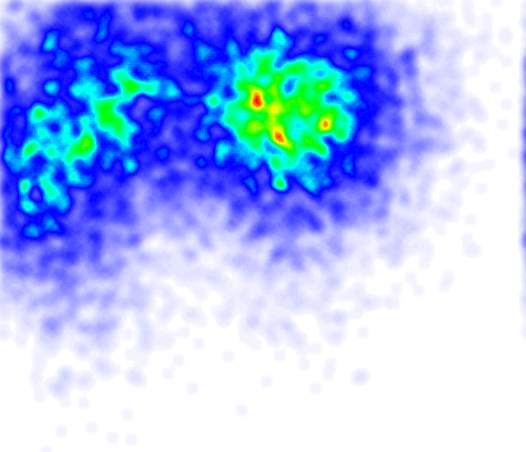# pyHeatMap：使用 Python 绘制热图的库

pyHeatMap 是一个使用 Python 生成热图的库，基本代码是我一年多之前写的，最近把它从项目中抠出来做成一个独立的库并开源。

``````pip install pyheatmap

# 或者

easy_install pyheatmap
``````

pyHeatMap 依赖于 PIL 库，使用之前需要先确保你的环境中已经安装了 PIL。使用方法很简单，比如：

``````# -*- coding: utf-8 -*-

import urllib
from pyheatmap.heatmap import HeatMap

def main():

# 下载测试数据
url = "https://raw.github.com/oldj/pyheatmap/master/examples/test_data.txt"
data = []
for ln in sdata:
a = ln.split(",")
if len(a) != 2:
continue
a = [int(i) for i in a]
data.append(a)

# 开始绘制
hm = HeatMap(data)
hm.clickmap(save_as="hit.png")
hm.heatmap(save_as="heat.png")

if __name__ == "__main__":
main()
````````````def example2():

hm = HeatMap(data_1)
hit_img = hm.clickmap()
hm2 = HeatMap(data_2)
hit_img2 = hm2.clickmap(base=hit_img, color=(0, 0, 255, 255))
hit_img2.save("hit2.png")
``````a